true
true

Ganesh WadkarDhanori, Pune, India - 411015Featured

# Ganesh Wadkar## Tutor

Dhanori, Pune, India - 411015.

2 Students taught

5.0

UrbanPro Rating
Referral Discount: Get ₹ 500 off when you make a payment to start classes. Get started by Booking a Demo.

Details verified of Ganesh Wadkar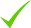IdentityEducation

Know how UrbanPro verifies Tutor details

Identity is verified based on matching the details uploaded by the Tutor with government databases.

## Overview

I am an experienced, qualified tutor with over 5 years of experience in teaching physics and maths.passionate about teaching physics concepts and maths numericals. I have good experience of competitive examinations like NDA, CDSE.

## Languages Spoken

Marathi Mother Tongue (Native)

English Proficient

Hindi Proficient

## Education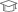fergusson 2013

Bachelor of Science (B.Sc.)pune university 2015

Master of Science (M.Sc.)

## Address

Dhanori, Pune, India - 411015

## Verified Info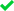ID VerifiedEducation VerifiedPhone VerifiedEmail Verified

Report this Profile

Is this listing inaccurate or duplicate? Any other problem?

Please tell us about the problem and we will fix it.

Please describe the problem that you see in this page.

Type the letters as shown below *

Please enter the letters as show below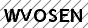Class 10 Tuition

Class LocationOnline (video chat via skype, google hangout etc)Student's HomeTutor's Home

Years of Experience in Class 10 Tuition

4

NIOS Subjects taught

Mathematics, Science

Board

CBSE, International Baccalaureate, State, ICSE, IGCSE, NIOS

State boards taught

Maharashtra State Board

IB Subjects taught

Physics, Mathematics

CBSE Subjects taught

Social science, Science, Mathematics

ICSE Subjects taught

Physics

IGCSE Subjects taught

Physics

Taught in School or College

Yes

State Syllabus Subjects taught

Science, Mathematics

## Reviews

this is test message this is test message this is test message this is test message this is test message this is test message this is test message

No Reviews yet! Be the first one to Review

## FAQs

1. Which school boards of Class 10 do you teach for?

CBSE, International Baccalaureate, State and others

2. Do you have any prior teaching experience?

Yes

3. Which classes do you teach?

I teach Class 10 Tuition, Class 11 Tuition, Class 12 Tuition, Class 7 Tuition, Class 8 Tuition, Class 9 Tuition and UPSC Exams Coaching Classes.

4. Do you provide a demo class?

Yes, I provide a paid demo class.

5. How many years of experience do you have?

I have been teaching for 4 years.

## Answers by Ganesh Wadkar (4)

Answered on 20/08/2019

You can guess the square root with simple logic. For example 81. - sq root is 9 361 is sq root of number whose unit = 1 or 9 Square of 20 is 400 .so u can it's square root of either 11 or 19. If you compare sq of 20 u can easily guess it sq root of 19. If last digit is 4 then it's square root of... ...more

You can guess the square root with simple logic.

For example

81.   - sq root is 9

361 is sq root of number whose unit = 1 or 9

Square of 20 is 400 .so u can it's square root of either 11 or 19.

If you compare sq of 20 u can easily guess it sq root of 19.

If last digit is 4 then it's square root of either 2 or 8

If last digit is 9 then it's square root of either 7 or 3

If last digit is 6 then it's square root of either 4 or 6

And if it's 5 then 5

Answers 73 Comments
Dislike Bookmark

Answered on 18/08/2019 CBSE/Class 9/Mathematics/Surface Area and Volumes/NCERT Solutions/Exercise 13.7

given h = 15 cm volume of cone = 1570 to find- radius (r) formula- volume of cone = 1570 = 3.14 * * 15 /3 1570 *3 = 3.14 * 15 * = 1570 * 3 / 3.14 * 15 =100 taking square roots on both sides , we get r = 10 cm ...more

given

h = 15 cm

volume of cone = 1570

to find- radius (r)

formula-

volume of cone =

1570     = 3.14 *  * 15 /3

1570 *3 =  3.14 * 15 *

=   1570 * 3 / 3.14 * 15

=100

taking square roots on both sides , we get

r = 10 cm

Answers 6 Comments
Dislike Bookmark

Answered on 18/08/2019 CBSE/Class 9/Mathematics/Surface Area and Volumes/NCERT Solutions/Exercise 13.7

Diameter of the conical pit = 3.5m radius= 1.75m height=12m volume of cone= π h /3 = 3.14 * 1.75^2 * 12 /3 =115.39/3 = 38.46 volume of cone = 38.46 m^3 1= 1000 L = 1 kilolitre hence , 38.5 = 38.5 kilolitrs ...more

Diameter of the conical pit = 3.5m

radius= 1.75m

height=12m

volume of cone= π h /3

= 3.14 * 1.75^2 * 12  /3

=115.39/3

= 38.46

volume of cone = 38.46 m^3

1= 1000 L = 1 kilolitre

hence ,

38.5  = 38.5 kilolitrs

Answers 3 Comments
Dislike Bookmark

Answered on 17/08/2019 CBSE/Class 9/Mathematics/Surface Area and Volumes/NCERT Solutions/Exercise 13.7

1. L^2 = h^2 + r^2 L = slant height h= height r= radius 25^2 = h^2 + 7^2 h^2 = 625 - 49 = 576 after taking square root h = 24 volume of cone = L^2 = h^2 + r^2 = 1/3 * 3.14 *7^2 *... ...more

1.    L^2 = h^2 + r^2              L = slant height   h= height  r= radius

25^2 = h^2 + 7^2

h^2 = 625 - 49 = 576

after taking square root

h = 24

volume of cone =                               L^2 = h^2 + r^2

= 1/3 * 3.14 *7^2 * 24

=3692.64/3

volume of cone  =1230 cm^3

1L = 1000cm^3

therefore answer is 1.230 L

Answers 3 Comments
Dislike Bookmark
Class 10 Tuition 5.0

Class LocationOnline (video chat via skype, google hangout etc)Student's HomeTutor's Home

Years of Experience in Class 10 Tuition

4

NIOS Subjects taught

Mathematics, Science

Board

CBSE, International Baccalaureate, State, ICSE, IGCSE, NIOS

State boards taught

Maharashtra State Board

IB Subjects taught

Physics, Mathematics

CBSE Subjects taught

Social science, Science, Mathematics

ICSE Subjects taught

Physics

IGCSE Subjects taught

Physics

Taught in School or College

Yes

State Syllabus Subjects taught

Science, Mathematics

Class 12 Tuition 5.0

Class Location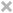Online (video chat via skype, google hangout etc)Student's HomeTutor's Home

Years of Experience in Class 12 Tuition

5

NIOS Subjects taught

Geography, Physics

Board

CBSE, State, NIOS, ISC/ICSE

ISC/ICSE Subjects taught

Physics

CBSE Subjects taught

Physics

Taught in School or College

No

State Syllabus Subjects taught

Mathematics, Physics

Class 9 Tuition 5.0

Class LocationOnline (video chat via skype, google hangout etc)Student's HomeTutor's Home

Years of Experience in Class 9 Tuition

5

Board

CBSE, State, ICSE, NIOS

CBSE Subjects taught

Science, Mathematics

ICSE Subjects taught

Physics

Taught in School or College

Yes

State Syllabus Subjects taught

Science, Mathematics

Class 11 Tuition 5.0

Class LocationOnline (video chat via skype, google hangout etc)Student's HomeTutor's Home

Years of Experience in Class 11 Tuition

5

NIOS Subjects taught

Geography, Mathematics, Physics

Board

CBSE, State, NIOS, ISC/ICSE

ISC/ICSE Subjects taught

Mathematics, Physics

CBSE Subjects taught

Physics, Mathematics

Taught in School or College

No

State Syllabus Subjects taught

Mathematics, History, Geography, Physics

Class 8 Tuition 5.0

Class LocationOnline (video chat via skype, google hangout etc)Student's HomeTutor's Home

Years of Experience in Class 8 Tuition

5

Board

CBSE, ICSE, State

CBSE Subjects taught

Mathematics, Science

ICSE Subjects taught

Physics, Chemistry, Mathematics

Experience in School or College

I have done my internship from reputed school and it was good experience. I am associated with coaching institute where I am teaching Physics for 11th and 12th std from last 4 years.

Taught in School or College

Yes

State Syllabus Subjects taught

Science, Mathematics

Class 7 Tuition 5.0

Class LocationOnline (video chat via skype, google hangout etc)Student's HomeTutor's Home

Years of Experience in Class 7 Tuition

5

Board

CBSE, ICSE, State

State boards taught

Maharashtra State Board

CBSE Subjects taught

Mathematics

ICSE Subjects taught

Physics, Mathematics

Taught in School or College

No

State Syllabus Subjects taught

Science, Mathematics

UPSC Exams Coaching classes 5.0

Class LocationOnline (video chat via skype, google hangout etc)Student's HomeTutor's Home

Years of Experience in UPSC Exams Coaching classes

4

Gives

CDS (Combined Defence Service) Exam Coaching, NDA (National Defence Acadamy and Naval Acadamy) Exam Coaching

this is test message this is test message this is test message this is test message this is test message this is test message this is test message

No Reviews yet! Be the first one to Review

## Answers by Ganesh Wadkar (4)

Answered on 20/08/2019

You can guess the square root with simple logic. For example 81. - sq root is 9 361 is sq root of number whose unit = 1 or 9 Square of 20 is 400 .so u can it's square root of either 11 or 19. If you compare sq of 20 u can easily guess it sq root of 19. If last digit is 4 then it's square root of... ...more

You can guess the square root with simple logic.

For example

81.   - sq root is 9

361 is sq root of number whose unit = 1 or 9

Square of 20 is 400 .so u can it's square root of either 11 or 19.

If you compare sq of 20 u can easily guess it sq root of 19.

If last digit is 4 then it's square root of either 2 or 8

If last digit is 9 then it's square root of either 7 or 3

If last digit is 6 then it's square root of either 4 or 6

And if it's 5 then 5

Answers 73 Comments
Dislike Bookmark

Answered on 18/08/2019 CBSE/Class 9/Mathematics/Surface Area and Volumes/NCERT Solutions/Exercise 13.7

given h = 15 cm volume of cone = 1570 to find- radius (r) formula- volume of cone = 1570 = 3.14 * * 15 /3 1570 *3 = 3.14 * 15 * = 1570 * 3 / 3.14 * 15 =100 taking square roots on both sides , we get r = 10 cm ...more

given

h = 15 cm

volume of cone = 1570

to find- radius (r)

formula-

volume of cone =

1570     = 3.14 *  * 15 /3

1570 *3 =  3.14 * 15 *

=   1570 * 3 / 3.14 * 15

=100

taking square roots on both sides , we get

r = 10 cm

Answers 6 Comments
Dislike Bookmark

Answered on 18/08/2019 CBSE/Class 9/Mathematics/Surface Area and Volumes/NCERT Solutions/Exercise 13.7

Diameter of the conical pit = 3.5m radius= 1.75m height=12m volume of cone= π h /3 = 3.14 * 1.75^2 * 12 /3 =115.39/3 = 38.46 volume of cone = 38.46 m^3 1= 1000 L = 1 kilolitre hence , 38.5 = 38.5 kilolitrs ...more

Diameter of the conical pit = 3.5m

radius= 1.75m

height=12m

volume of cone= π h /3

= 3.14 * 1.75^2 * 12  /3

=115.39/3

= 38.46

volume of cone = 38.46 m^3

1= 1000 L = 1 kilolitre

hence ,

38.5  = 38.5 kilolitrs

Answers 3 Comments
Dislike Bookmark

Answered on 17/08/2019 CBSE/Class 9/Mathematics/Surface Area and Volumes/NCERT Solutions/Exercise 13.7

1. L^2 = h^2 + r^2 L = slant height h= height r= radius 25^2 = h^2 + 7^2 h^2 = 625 - 49 = 576 after taking square root h = 24 volume of cone = L^2 = h^2 + r^2 = 1/3 * 3.14 *7^2 *... ...more

1.    L^2 = h^2 + r^2              L = slant height   h= height  r= radius

25^2 = h^2 + 7^2

h^2 = 625 - 49 = 576

after taking square root

h = 24

volume of cone =                               L^2 = h^2 + r^2

= 1/3 * 3.14 *7^2 * 24

=3692.64/3

volume of cone  =1230 cm^3

1L = 1000cm^3

therefore answer is 1.230 L

Answers 3 Comments
Dislike Bookmark

Ganesh Wadkar describes himself as Tutor. He conducts classes in Class 10 Tuition, Class 11 Tuition and Class 12 Tuition. Ganesh is located in Dhanori, Pune. Ganesh takes at students Home and Regular Classes- at his Home. He has 5 years of teaching experience . Ganesh has completed Bachelor of Science (B.Sc.) from fergusson in 2013 and Master of Science (M.Sc.) from pune university in 2015. He is well versed in English, Hindi and Marathi.

•• Want to learn from Ganesh Wadkar?

• Contact Now
X

Share this Profile

Recommended Profiles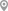Dhanori, PunePatel Nagar, DelhiDhanori, PuneDhanori, PuneKharadi, PuneNanded, Pune

### Learn More

X

Post your Learning Need

Let us shortlist and give the best tutors and institutes.

or

Send Enquiry to Ganesh Wadkar

Let Ganesh Wadkar know you are interested in their class

Reply to 's review

Enter your reply*

1500/1500

Please enter your reply

Your reply should contain a minimum of 10 charactersYour reply has been successfully submitted.

UrbanPro.com is India's largest network of most trusted tutors and institutes. Over 55 lakh students rely on UrbanPro.com, to fulfill their learning requirements across 1,000+ categories. Using UrbanPro.com, parents, and students can compare multiple Tutors and Institutes and choose the one that best suits their requirements. More than 7.5 lakh verified Tutors and Institutes are helping millions of students every day and growing their tutoring business on UrbanPro.com. Whether you are looking for a tutor to learn mathematics, a German language trainer to brush up your German language skills or an institute to upgrade your IT skills, we have got the best selection of Tutors and Training Institutes for you. Read more